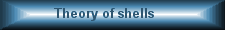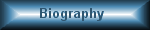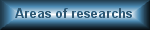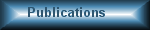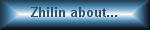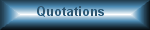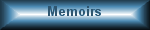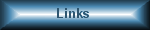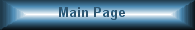Pavel Zhilin
 Main   Biography   Photo   Areas of reseaches   Publications   Zhilin about...   Quotations   Memoirs   Links
 The range of scientific interests of P.A. Zhilin is very wide. It includes fundamental laws of mechanics, direct tensor calculus, rigid body dynamics, nonlinear rod theory, general theory of inelastic media, including plasticity, consolidating granular media, phase transitions, as well as piezoelectricity, ferromagnetism, electrodynamics and quantum mechanics. At first sight it seems that the investigations are not related one to another. But this is not so. Let us show a few examples. Rigid body oscillator and gyrostat on an elastic foundation, which mathematical description concerns to area of the rigid body dynamics, are used further as fundamental model when constructing inelastic media theory, piezoelectricity theory, theory of magnetoelastic materials, and also for derivation of Maxwell's equations. Methods of description of the spinor motion, based on use of the direct tensor calculus, are used and developed both for solving rigid body dynamics problems and for solving nonlinear rod theory problems. The same methods are used when constructing various continuum models, which take into account rotational degrees of freedom: shells, rods, plastic materials, consolidating granular materials, piezoelectric materials, magnetoelastic materials, and electromagnetic field. The symmetry theory and tensor invariant theory are being actively used and developed when constructing shell theory, rod theory, as well as for other continuum theories: general theory of inelastic media, piezoelectricity theory and ferromagnetism theory. The huge attention is given base concepts and fundamental laws of mechanics. Some papers are devoted to a consecutive statement of fundamental laws of the Eulerian mechanics — mechanics of bodies of a general kind, consisting of particles with rotational degrees of freedom. All continuum theories developed by P.A.Zhilin, including models, which mathematical description, finally, is reduced to Maxwell's equations, Schrödinger's equation and Klein-Gordon's equation, are constructed adhering to the same positions based on the fundamental laws of mechanics. When constructing continuum models both for elastic (shells, rods, piezoelectric materials, magnetoelastic materials, electromagnetic field) and inelastic media, the theory of strains, proposed by P.A. Zhilin, is used. The theory of strains is based on the idea of using the reduced energy balance equation for defining measures of deformation. By the elementary examples of discrete systems mechanics the concept of internal energy, chemical potential, temperature and entropy are introduced. Definition of these quantities is given by means of pure mechanical arguments, which are based on using special mathematical formulation of energy balance equation. The same method of introducing the basic concepts of thermodynamics is used when constructing continuum theories. In fact P.A. Zhilin's papers represent the method for constructing continuum theories with rotational degrees of freedom together with the necessary mathematical technique, as well as examples of using the mentioned theories when describing different physical phenomena, including phenomena which are considered not subject to classical mechanics.